# Fixed-Structure H-infinity Synthesis with `hinfstruct`

This example uses the `hinfstruct` command to tune a fixed-structure controller subject to ${H}_{\infty }$ constraints.

### Introduction

The `hinfstruct` command extends classical ${H}_{\infty }$ synthesis (see `hinfsyn`) to fixed-structure control systems. This command is meant for users already comfortable with the `hinfsyn` workflow. If you are unfamiliar with ${H}_{\infty }$ synthesis or find augmented plants and weighting functions intimidating, use `systune` and `looptune` instead. See Tuning Control Systems with SYSTUNE for the `systune` counterpart of this example.

### Plant Model

This example uses a 9th-order model of the head-disk assembly (HDA) in a hard-disk drive. This model captures the first few flexible modes in the HDA.

```load hinfstruct_demo G bode(G), grid```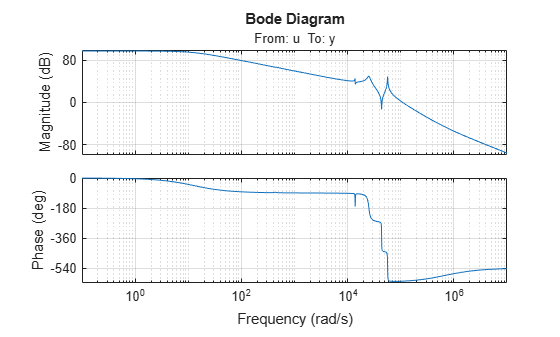We use the feedback loop shown below to position the head on the correct track. This control structure consists of a PI controller and a low-pass filter in the return path. The head position `y` should track a step change `r` with a response time of about one millisecond, little or no overshoot, and no steady-state error.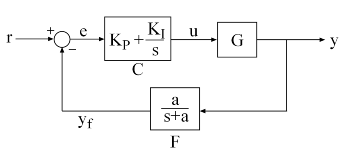Figure 1: Control Structure

### Tunable Elements

There are two tunable elements in the control structure of Figure 1: the PI controller $C\left(s\right)$ and the low-pass filter

`$F\left(s\right)=\frac{a}{s+a}.$`

Use the `tunablePID` class to parameterize the PI block and specify the filter $F\left(s\right)$ as a transfer function depending on a tunable real parameter $a$.

```C0 = tunablePID('C','pi'); % tunable PI a = realp('a',1); % filter coefficient F0 = tf(a,[1 a]); % filter parameterized by a```

### Loop Shaping Design

Loop shaping is a frequency-domain technique for enforcing requirements on response speed, control bandwidth, roll-off, and steady state error. The idea is to specify a target gain profile or "loop shape" for the open-loop response $L\left(s\right)=F\left(s\right)G\left(s\right)C\left(s\right)$. A reasonable loop shape for this application should have integral action and a crossover frequency of about 1000 rad/s (the reciprocal of the desired response time of 0.001 seconds). This suggests the following loop shape:

```wc = 1000; % target crossover s = tf('s'); LS = (1+0.001*s/wc)/(0.001+s/wc); bodemag(LS,{1e1,1e5}), grid, title('Target loop shape')```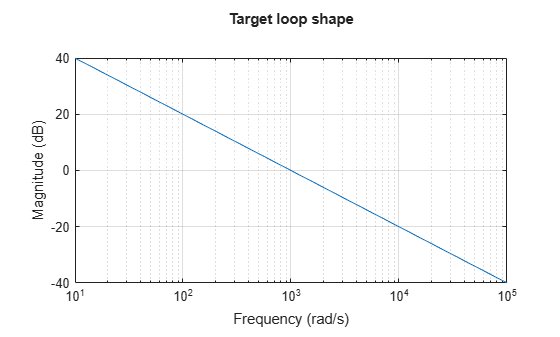Note that we chose a bi-proper, bi-stable realization to avoid technical difficulties with marginally stable poles and improper inverses. In order to tune $C\left(s\right)$ and $F\left(s\right)$ with `hinfstruct`, we must turn this target loop shape into constraints on the closed-loop gains. A systematic way to go about this is to instrument the feedback loop as follows:

• Add a measurement noise signal `n`

• Use the target loop shape `LS` and its reciprocal to filter the error signal `e` and the white noise source `nw`.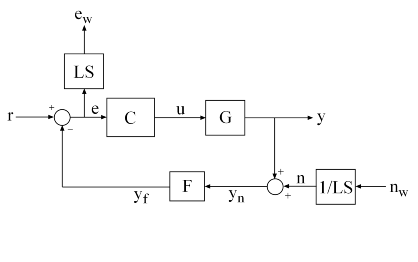Figure 2: Closed-Loop Formulation

If $T\left(s\right)$ denotes the closed-loop transfer function from `(r,nw)` to `(y,ew)`, the gain constraint

`$‖T{‖}_{\infty }<1$`

secures the following desirable properties:

• At low frequency (w<wc), the open-loop gain stays above the gain specified by the target loop shape `LS`

• At high frequency (w>wc), the open-loop gain stays below the gain specified by `LS`

• The closed-loop system has adequate stability margins

• The closed-loop step response has small overshoot.

We can therefore focus on tuning $C\left(s\right)$ and $F\left(s\right)$ to enforce $‖T{‖}_{\infty }<1$.

### Specifying the Control Structure in MATLAB

In MATLAB, you can use the `connect` command to model $T\left(s\right)$ by connecting the fixed and tunable components according to the block diagram of Figure 2:

```% Label the block I/Os Wn = 1/LS; Wn.u = 'nw'; Wn.y = 'n'; We = LS; We.u = 'e'; We.y = 'ew'; C0.u = 'e'; C0.y = 'u'; F0.u = 'yn'; F0.y = 'yf'; % Specify summing junctions Sum1 = sumblk('e = r - yf'); Sum2 = sumblk('yn = y + n'); % Connect the blocks together T0 = connect(G,Wn,We,C0,F0,Sum1,Sum2,{'r','nw'},{'y','ew'});```

These commands construct a generalized state-space model `T0` of $T\left(s\right)$. This model depends on the tunable blocks `C` and `a`:

`T0.Blocks`
```ans = struct with fields: C: [1x1 tunablePID] a: [1x1 realp] ```

Note that `T0` captures the following "Standard Form" of the block diagram of Figure 2 where the tunable components $C,F$ are separated from the fixed dynamics.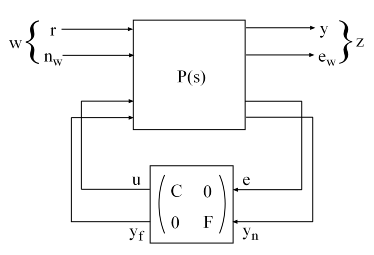Figure 3: Standard Form for Disk-Drive Loop Shaping

### Tuning the Controller Gains

We are now ready to use `hinfstruct` to tune the PI controller $C$ and filter $F$ for the control architecture of Figure 1. To mitigate the risk of local minima, run three optimizations, two of which are started from randomized initial values for `C0` and `F0`:

```rng('default') opt = hinfstructOptions('Display','final','RandomStart',5); T = hinfstruct(T0,opt);```
```Final: Peak gain = 3.88, Iterations = 67 Final: Peak gain = 597, Iterations = 188 Some closed-loop poles are marginally stable (decay rate near 1e-07) Final: Peak gain = 597, Iterations = 167 Some closed-loop poles are marginally stable (decay rate near 1e-07) Final: Peak gain = 3.88, Iterations = 66 Final: Peak gain = 1.56, Iterations = 106 Final: Peak gain = 1.56, Iterations = 93 ```

The best closed-loop gain is 1.56, so the constraint $‖T{‖}_{\infty }<1$ is nearly satisfied. The `hinfstruct` command returns the tuned closed-loop transfer $T\left(s\right)$. Use `showTunable` to see the tuned values of $C$ and the filter coefficient $a$:

`showTunable(T)`
```C = 1 Kp + Ki * --- s with Kp = 0.000846, Ki = 0.0103 Name: C Continuous-time PI controller in parallel form. ----------------------------------- a = 5.49e+03 ```

Use `getBlockValue` to get the tuned value of $C\left(s\right)$ and use `getValue` to evaluate the filter $F\left(s\right)$ for the tuned value of $a$:

```C = getBlockValue(T,'C'); F = getValue(F0,T.Blocks); % propagate tuned parameters from T to F tf(F)```
```ans = From input "yn" to output "yf": 5486 -------- s + 5486 Continuous-time transfer function. ```

To validate the design, plot the open-loop response `L=F*G*C` and compare with the target loop shape `LS`:

```bode(LS,'r--',G*C*F,'b',{1e1,1e6}), grid, title('Open-loop response'), legend('Target','Actual')```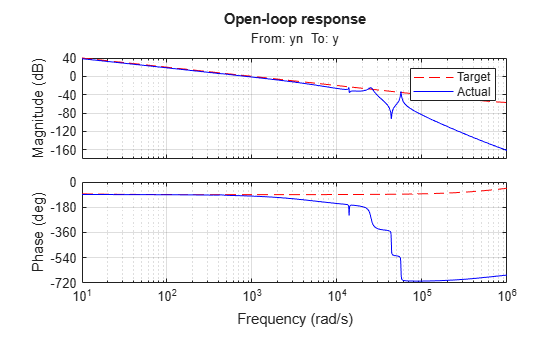The 0dB crossover frequency and overall loop shape are as expected. The stability margins can be read off the plot by right-clicking and selecting the Characteristics menu. This design has 24dB gain margin and 81 degrees phase margin. Plot the closed-loop step response from reference `r` to position `y`:

`step(feedback(G*C,F)), grid, title('Closed-loop response')`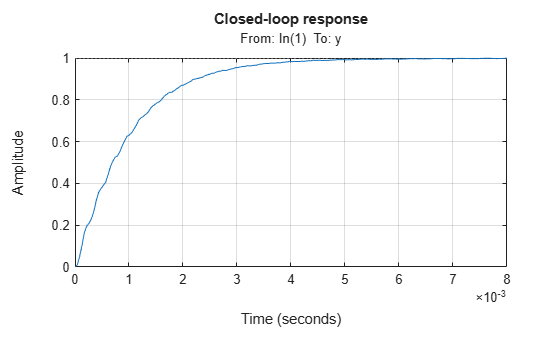While the response has no overshoot, there is some residual wobble due to the first resonant peaks in `G`. You might consider adding a notch filter in the forward path to remove the influence of these modes.

### Tuning the Controller Gains from Simulink

Suppose you used this Simulink model to represent the control structure. If you have Simulink Control Design installed, you can tune the controller gains from this Simulink model as follows. First mark the signals `r,e,y,n` as Linear Analysis points in the Simulink model.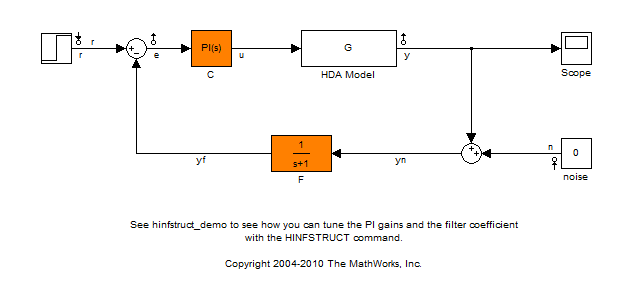Then create an instance of the `slTuner` interface and mark the Simulink blocks `C` and `F` as tunable:

`ST0 = slTuner('rct_diskdrive',{'C','F'});`

Since the filter $F\left(s\right)$ has a special structure, explicitly specify how to parameterize the `F` block:

```a = realp('a',1); % filter coefficient setBlockParam(ST0,'F',tf(a,[1 a]));```

Finally, use `getIOTransfer` to derive a tunable model of the closed-loop transfer function $T\left(s\right)$ (see Figure 2)

```% Compute tunable model of closed-loop transfer (r,n) -> (y,e) T0 = getIOTransfer(ST0,{'r','n'},{'y','e'}); % Add weighting functions in n and e channels T0 = blkdiag(1,LS) * T0 * blkdiag(1,1/LS);```

You are now ready to tune the controller gains with `hinfstruct`:

```rng(0) opt = hinfstructOptions('Display','final','RandomStart',5); T = hinfstruct(T0,opt);```
```Final: Peak gain = 3.88, Iterations = 67 Final: Peak gain = 597, Iterations = 192 Some closed-loop poles are marginally stable (decay rate near 1e-07) Final: Peak gain = 596, Iterations = 175 Some closed-loop poles are marginally stable (decay rate near 1e-07) Final: Peak gain = 3.88, Iterations = 70 Final: Peak gain = 1.56, Iterations = 102 Final: Peak gain = 1.56, Iterations = 99 ```

Verify that you obtain the same tuned values as with the MATLAB approach:

`showTunable(T)`
```C = 1 Kp + Ki * --- s with Kp = 0.000846, Ki = 0.0103 Name: C Continuous-time PI controller in parallel form. ----------------------------------- a = 5.49e+03 ```